# Can I calculate values (formulas alternative)?

### When would I need calculations or formulas?

Sometimes you may want to calculate a column value based on other data in the row, for example:

• If we are tracking orders and have columns for Price and Amount, we might want to calculate the Order Total, which should equal Price x Amount.
• If we are tracking project deadlines and have columns for Start Date and Effort, we might want to calculate the End Date by taking the Start Date and adding the number of days in Effort.
• If we are tracking a sales pipeline and have columns for the sale Stage and Deal Amount, we might want to add a weekly snapshot of the total deal amount per stage.

These types of use cases are typical examples of when you'd want to use some calculations, and in spreadsheets you would traditionally use formulas to accomplish this.

### How to do calculations in Tables

We do not have a native calculation column type for this yet; however, you can do this using our Apps Script bot action and the below sample script code to pretty easily (and flexibly) set up and customize your own calculation logic.

### 1. Setting up the table

The setup for using the bot action is quite simple! We recommend having a table with at least 2 to 3 columns:

1. A first column to store the first value, this could be a Number-type column called "`Price`".
2. If you want to modify the first value using a second value, for example, to add or multiple two numbers together, you will need a second column to store the second value. This could be another Number-type column called "`Amount`".
3. Finally a third column to store the final result of the calculation, this can be a Number-type column called "`Total`".

Once you have your table setup with the right columns and data, we can go over to Apps Script to quickly put together the script to do the calculation, and then finally call the script from our bot action.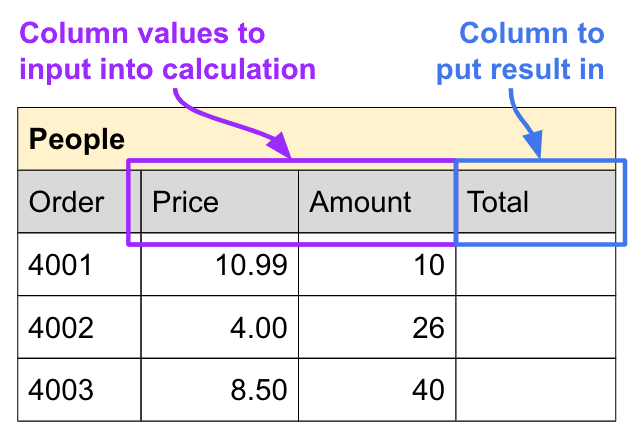### 2. Setting up Apps Script function

1. Open script.google.com to access the Apps Script project list.
2. Create a new Apps Script project and give it a recognizable project name (so you can find it when selecting the script for your bot action later).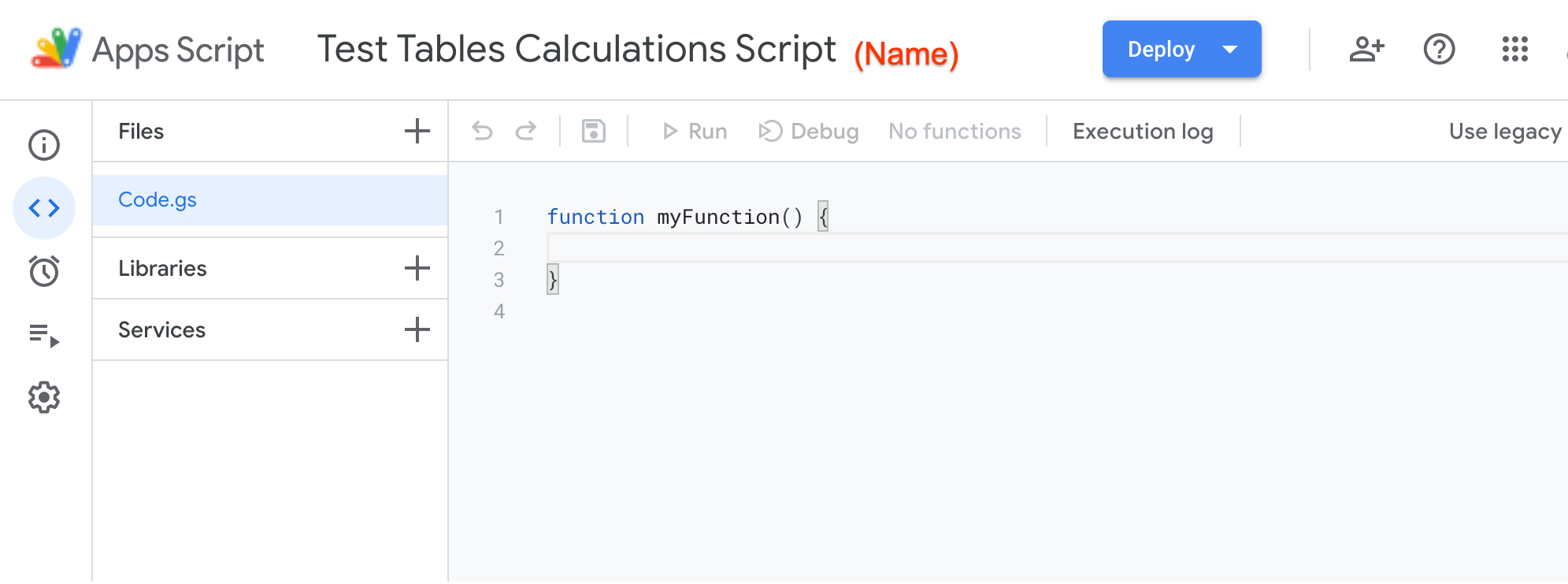3. Ensure you have the Tables API service enabled.
4. Then copy and paste any combination of the following code snippets below into your script to use:
NOTE: column names are case-sensitive, we will get the `tableId` and `rowId` from bot variables.

## Numbers

```/**   * ADDS two number column values together and saves into a column  * @param tableId - id of the table to read row data from  * @param rowId - id of the row to read data from and update  * @param firstColName - name of column with first value  * @param secondColName - name of column with second value  * @param resultColName - name of column to store calculated value  */ function add(tableId, rowId, firstColName, secondColName, resultColName) {   // Get the row of data from the table, make sure it exists or abort   const row = Area120Tables.Tables.Rows.get('tables/' + tableId + '/rows/' + rowId);   if (!row) { console.error('Row does not exist'); return; }```

```  // Do the calculation with the values from the columns   ``````const firstValue = (row.values[firstColName] || 0);   const secondValue = (row.values[secondColName] || 0);   const result = firstValue + secondValue;```

```  // Save the new calculated value to the result column   row.values[resultColName] = result;   Area120Tables.Tables.Rows.patch(row, row.name); }```

## Subtract two Number-type columns

```/**   * SUBTRACTS two number column values and saves into a column  * @param tableId - id of the table to read row data from  * @param rowId - id of the row to read data from and update  * @param baseColName - name of column with value to start with  * @param subtractColName - name of column with value to subtract  * @param resultColName - name of column to store calculated value  */ function subtract(tableId, rowId, baseColName, subtractColName, resultColName) {   // Get the row of data from the table, make sure it exists or abort   const row = Area120Tables.Tables.Rows.get('tables/' + tableId + '/rows/' + rowId);   if (!row) { console.error('Row does not exist'); return; }```

```  // Do the calculation with the values from the columns   const baseValue = (row.values[baseColName] || 0);   const subtractValue = (row.values[subtractColName] || 0);   const result = baseValue - subtractValue;```

```  // Save the new calculated value to the result column   row.values[resultColName] = result;   Area120Tables.Tables.Rows.patch(row, row.name); }```

## Multiply two Number-type columns

```/**   * MULTIPLIES two number column values and saves into a column  * @param tableId - id of the table to read row data from  * @param rowId - id of the row to read data from and update  * @param firstColName - name of column with first value  * @param secondColName - name of column with second value  * @param resultColName - name of column to store calculated value  */```

```function multiply(tableId, rowId, firstColName, secondColName, resultColName) {   // Get the row of data from the table, make sure it exists or abort   const row = Area120Tables.Tables.Rows.get('tables/' + tableId + '/rows/' + rowId);   if (!row) { console.error('Row does not exist'); return; }```

```  // Do the calculation with the values from the columns   const firstValue = (row.values[firstColName] || 0);   const secondValue = (row.values[secondColName] || 0);   const result = firstValue * secondValue;```

```  // Save the new calculated value to the result column   row.values[resultColName] = result;   Area120Tables.Tables.Rows.patch(row, row.name); }```

## Divide two Number-type columns

```/**   * DIVIDES two number column values and saves into a column  * @param tableId - id of the table to read row data from  * @param rowId - id of the row to read data from and update  * @param baseColName - name of column with value to start with  * @param divisorColName - name of column with value to divide by  * @param resultColName - name of column to store calculated value  */```
```function divide(tableId, rowId, baseColName, divisorColName, resultColName) {   // Get the row of data from the table, make sure it exists or abort   const row = Area120Tables.Tables.Rows.get('tables/' + tableId + '/rows/' + rowId);   if (!row) { console.error('Row does not exist'); return; }```

```  // Do the calculation with the values from the columns   const baseValue = (row.values[baseColName] || 0);   const divideValue = row.values[divisorColName];   if (!divideValue) { console.error('No divisor value'); return; }   const result = baseValue / divideValue;```

```  // Save the new calculated value to the result column   row.values[resultColName] = result;   Area120Tables.Tables.Rows.patch(row, row.name); }```

## Add # of days to a Date-type column

```/**   * ADDS days to a date column value and saves into a column  * Note: also works with negative values to go back days  * @param tableId - id of the table to read row data from  * @param rowId - id of the row to read data from and update  * @param dateColName - name of column with the date value  * @param numberColName - name of column with # of days to add  * @param resultColName - name of column to store calculated value  */```
```function date_add(tableId, rowId, dateColName, numberColName, resultColName) {   // Get the row of data from the table, make sure it exists or abort   const row = Area120Tables.Tables.Rows.get('tables/' + tableId + '/rows/' + rowId);   if (!row) { console.error('Row does not exist'); return; }   // Helper function to convert common types to date   function toDate(val) {     if (typeof val === 'object' && val !== null) {       if (val.seconds) { return new Date(val.seconds * 1000); }       if (val.year)    { return new Date(val.year, val.month-1, val.day); }     }     return new Date(val);   }```

```  // Do the calculation with the values from the columns   const date = toDate(row.values[dateColName]);   const daysToAdd = row.values[numberColName];   date.setDate(date.getDate() + daysToAdd);```

```  // Save the new calculated value to the result column   row.values[resultColName] = date;   Area120Tables.Tables.Rows.patch(row, row.name); }```

## Subtract two Date-type columns to get # of days between

```/**   * SUBTRACTS two date column values to calculate # of days  * between the two dates, and saves into a column  * @param tableId - id of the table to read row data from  * @param rowId - id of the row to read data from and update  * @param baseColName - name of column with date to start with  * @param diffColName - name of column with date to subtract  * @param resultColName - name of column to store calculated value  */```
```function date_dif(tableId, rowId, dateColName, diffColName, resultColName) {   // Get the row of data from the table, make sure it exists or abort   const row = Area120Tables.Tables.Rows.get('tables/' + tableId + '/rows/' + rowId);   if (!row) { console.error('Row does not exist'); return; }   // Helper function to convert common types to date   function toDate(val) {     if (typeof val === 'object' && val !== null) {       if (val.seconds) { return new Date(val.seconds * 1000); }       if (val.year)    { return new Date(val.year, val.month-1, val.day); }     }```
```    return new Date(val);   }```

```  // Do the calculation with the values from the columns   const oneDay = 24 * 60 * 60 * 1000; // # of milliseconds in a day   const firstDate = toDate(row.values[dateColName]);   const secondDate = toDate(row.values[diffColName]);   const diffDays = Math.round(Math.abs((firstDate - secondDate) / oneDay));```

```  // Save the new calculated value to the result column   row.values[resultColName] = diffDays;   Area120Tables.Tables.Rows.patch(row, row.name); }```

## Concatenate two column values as text

```/**   * CONCATENATES two column values together as text and saves into a column  * @param tableId - id of the table to read row data from  * @param rowId - id of the row to read data from and update  * @param firstColName - name of column with first value  * @param secondColName - name of column with second value  * @param resultColName - name of column to store calculated value  */```
```function concat(tableId, rowId, firstColName, secondColName, resultColName) {   // Get the row of data from the table, make sure it exists or abort   const row = Area120Tables.Tables.Rows.get('tables/' + tableId + '/rows/' + rowId);   if (!row) { console.error('Row does not exist'); return; }```

```  // Helper function to handle special types   function toText(val) {     if (typeof val === 'object' && val !== null) {       if (val.address) { return val.address; }       if (val.seconds) { return new Date(val.seconds * 1000); }``````       if (val.year)    { return new Date(val.year, val.month-1, val.day); }     }     if (Array.isArray(val)) {       if (!val.length) { return ''; }       const item = val;       if (item.url) { return val.map(i => i.url).join(', '); }       else { return val.join(', '); }     }     return val;   }```

```  // Do the calculation with the values from the columns   const firstValue = toText(row.values[firstColName]);   const secondValue = toText(row.values[secondColName]);   const result = '' + firstValue + secondValue;```

```  // Save the new calculated value to the result column   row.values[resultColName] = result;   Area120Tables.Tables.Rows.patch(row, row.name); }```

## Calculate character length of a Text-type column

```/**   * Calculates the length of text and saves in a number column  * @param tableId - id of the table to read row data from  * @param rowId - id of the row to read data from and update  * @param textColName - name of column with text value  * @param resultColName - name of number column to store text length  */```
```function length(tableId, rowId, textColName, resultColName) {   // Get the row of data from the table, make sure it exists or abort   const row = Area120Tables.Tables.Rows.get('tables/' + tableId + '/rows/' + rowId);   if (!row) { console.error('Row does not exist'); return; }```

```  // Do the calculation with the values from the columns   const value = row.values[textColName];   const result = value && value.length ? value.length : 0;```

```  // Save the new calculated value to the result column   row.values[resultColName] = result;   Area120Tables.Tables.Rows.patch(row, row.name); }```

## Get matches to a regular expression for a Text-type column

```/**   * Gets matches to a dynamic regular expression for a text   * column, and saves the results into a text column  * @param tableId - id of the table to read row data from  * @param rowId - id of the row to read data from and update  * @param baseColName - name of column with text to search  * @param regexString - regular expression to match  * @param resultColName - name of column to store calculated value```
``` * @param regexFlags - (optional) advanced flags for regular expression  * Try regex101.com to test your regex,   */```
```function regex_match(tableId, rowId, baseColName, regexString, resultColName, regexFlags) {   // Get the row of data from the table, make sure it exists or abort   const row = Area120Tables.Tables.Rows.get('tables/' + tableId + '/rows/' + rowId);   if (!row) { console.error('Row does not exist'); return; }```

```  // Create and ensure valid regular expression   const regex = new RegExp(regexString, regexFlags);   if (!regex) { console.error('Invalid regex string'); return; };```

```  // Do the calculation with the values from the columns   const textValue = row.values[baseColName];   const matches = textValue.match(regex);   const result = matches ? matches.join(', ') : '';```

```  // Save the new calculated value to the result column   row.values[resultColName] = result;   Area120Tables.Tables.Rows.patch(row, row.name); }```

## Replace text with a regular expression for a Text-type column

```/**   * Replaces parts of text based on a dynamic regular expression  * for a text column, and saves the results into a text column  * @param tableId - id of the table to read row data from  * @param rowId - id of the row to read data from and update  * @param baseColName - name of column with text to search  * @param regexString - regular expression to replace  * @param replaceText - text to replace with  * @param resultColName - name of column to store calculated value```
``` * @param regexFlags - (optional) advanced flags for regular expression  * Try regex101.com to test your regex,   */```
```function regex_replace(tableId, rowId, baseColName, regexString, replaceText, resultColName, regexFlags) {   // Get the row of data from the table, make sure it exists or abort   const row = Area120Tables.Tables.Rows.get('tables/' + tableId + '/rows/' + rowId);   if (!row) { console.error('Row does not exist'); return; }```

```  // Create and ensure valid regular expression   const regex = new RegExp(regexString, regexFlags);   if (!regex) { console.error('Invalid regex string'); return; };```

```  // Do the calculation with the values from the columns   const textValue = row.values[baseColName];   const result = textValue.match(regex).join(', ');```

```  // Save the new calculated value to the result column   row.values[resultColName] = diffDays;   Area120Tables.Tables.Rows.patch(row, row.name); }```

## Run a calculation over each row in the table

This is a more advanced use case, for situations like:

• If you need to recalculate a lot of data in existing rows due to a change in the logic.
• On a time interval, you need all the rows in the table updated with new values in a column.

#### Apps Script code

```/**   * BULK CALCULATION script that runs and updates values for   * every row in the table. This works by passing in the table ID,   * the name of the bulk calculation function to use, and the   * parameters for the bulk calculation function. See the sample  * bulk calculation function at the bottom of this script.  *   * NOTE: BE CAREFUL HOW YOU USE THIS WITH TIME-BASED TRIGGERS,   * you can end up executing the entire script 100 times for   * 100 rows, if you don't set the right bot filters/conditions.  *   * @param tableId - id of the table to read row data from  * @param calcFunctionName - name of bulk calculation function  *   to use, this needs to match exactly  * @param ... in your bot, you should continue to add more params  *   that you will use for your calculation function, this will  *   be accessed via a special auto-generated "arguments" property.  */ const SCRIPT = this;    // Save this for calling function later const page_size = 500;  // Max page size for update call to API ```
```function bulkRowCalculation(tableId, calcFunctionName) {   console.log('params:', tableId, calcFunctionName, arguments);   if (!tableId) { console.error('TableId does not exist'); return; }   const tableName = 'tables/' + tableId;```

```  // Get a reference to the calculation function by the function name   // and prep the parameters to pass to the calculation function.   // Remove first two params in the "arguments", since those two are   // tableId and calcFunctionName from the original function call   const calcFunction = SCRIPT[calcFunctionName];   const params = [...arguments].slice(2);```

```  // Get first page of rows from the table   let response = Area120Tables.Tables.Rows.list(tableName, {page_size});   let page_token, pageCount = 1;  ```

```  while (response) {  // Loop through pages until no more pages     const rows = response.rows;     console.log('pageNum: ' + pageCount, 'numRows: ' + rows.length);```

```    // Process rows with calculation function, pass along the row     // that we're processing, as well as the extra parameters     for (let row of rows) {       calcFunction.apply(this, [row, ...params]);     }```

```    // Send request to batch update all the processed rows     while requests = rows.map(row => { return {row}; });     Area120Tables.Tables.Rows.batchUpdate({requests}, tableName);```

```    // Read next page of rows, if there are any more pages to read     page_token = response.nextPageToken;     response = page_token ? Area120Tables.Tables.Rows.list(tableName, {page_size, page_token}) : undefined;     pageCount++;   }```
`}`

```/**   * A sample bulk calculation function for use with bulkRowCalculation()  * This function is similar to many other sample scripts like "add()"   * and "multiply()", but with three key changes:  *  1. we do not pass in tableId and rowId, replace with single "row" param  *  2. we do not call the API to get the row, it's already passed in as  *     a parameter, so you can remove those lines  *  3. we do not call the API to update the row, that is handled later  *   * In the Tables bot, you will pass along the additional parameters  * for this calculation function after the tableId and calcFunctionName.```
``` *   * You should update the function name and parameters and the calculation  * logic to match what you want and need.  */```
```function sampleBulkCalcFunction(row, firstColName, secondColName, resultColName) {   if (!row) { console.error('Row does not exist'); return; }```

```  // Do the calculation with the values from the columns   const firstValue = (row.values[firstColName] || 0);   const secondValue = (row.values[secondColName] || 0);   const result = firstValue * secondValue;  // Multiply```

```  // Save the new calculated value to the result column   row.values[resultColName] = diffDays; }```

#### Tables bot setup

In the bot action parameters, you will want to pass along the table's ID, the name of the bulk calculation function you want to use, and then the parameters you will need for your bulk calculation function.

This is what your Apps Script bot action parameters may look like to use the above script correctly, if you were trying to use the `sampleBulkCalcFunction()` to multiple your Price column with Amount column to get the total Cost:

1. [[table_id]]
2. sampleBulkCalcFunction
3. Price
4. Amount
5. Cost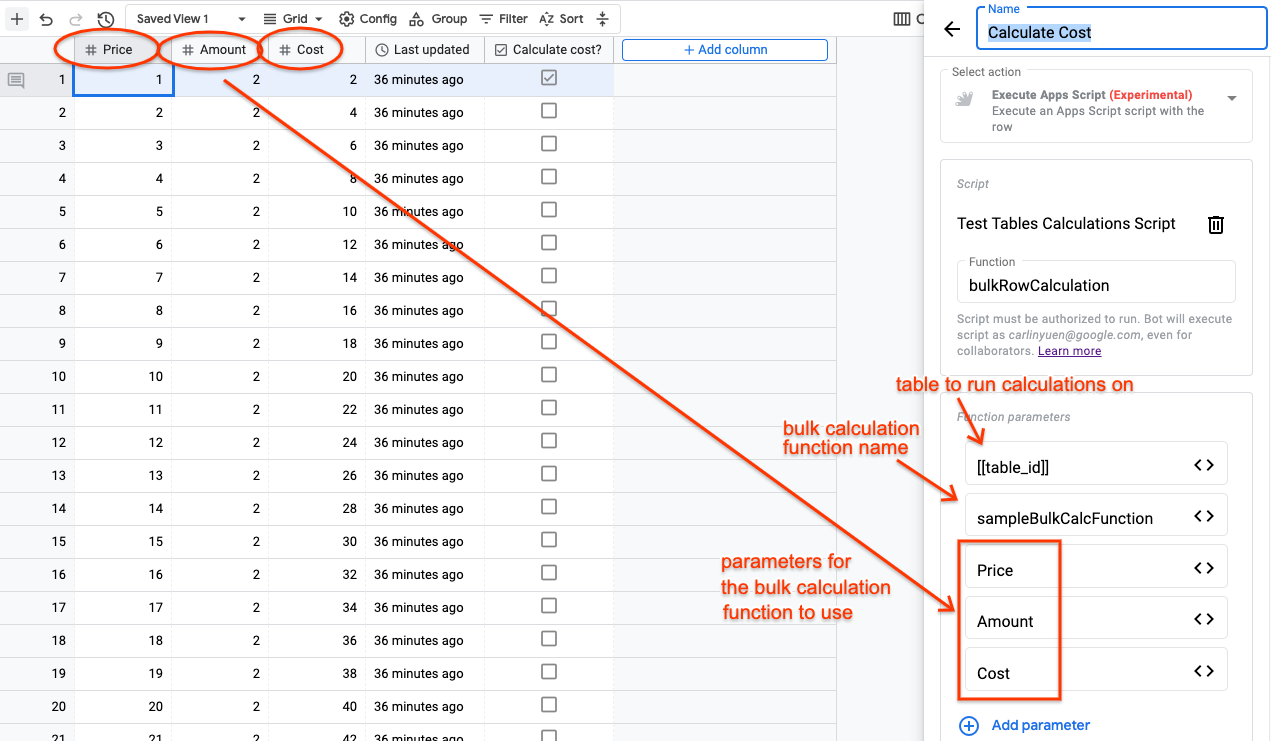## Calculate an aggregate value across all rows in the table

This is a more advanced use case, for situations like:

• If you need to calculate a running sum or count of column values across all rows in the table.
• Every week, you want to calculate the sum of all sales completed, or count all tasks done, and save it as a row in another table for a snapshot of historical performance.

This type of logic will require you to do some scripting to adjust the logic to match what business need you have.

#### Apps Script code

```/**   * AGGREGATE CALCULATION script that runs and does a running  * aggregation of some calculated value across all the rows  * in the table, and then saves it as a new row in a table.  * Because the calculation logic and the aggregations need to  * be very customized to your use case, we will only pass the  * tableId and the rest of the logic must be handled in the  * script and adjusted to your needs.   *   * Below is just an example of what you can do. In this case,  * we will calculate a count and sum the total deal value   * of deals in a sales pipeline by stage. This lets you track  * the progress in a sales pipeline over time.  *   * NOTE: BE CAREFUL HOW YOU USE THIS WITH TIME-BASED TRIGGERS,   * you can end up executing the entire script 100 times for   * 100 rows, if you don't set the right bot filters/conditions.  *   * @param tableId - id of the table to read row data from  */```
```function aggregateCalculation(tableId) {   console.log('tableId:', tableId);   if (!tableId) { console.error('TableId does not exist'); return; }   const tableName = 'tables/' + tableId;```

```  // Setup for aggregate calculations, adjust this as needed   const dateToday = new Date();   const date7DaysAgo = new Date().setDate(dateToday.getDate() - 7);   const values = {};  // Track calculated values   const toDate = function(val) { // Function to convert types to date     if (typeof val === 'object' && val !== null) {       if (val.seconds) { return new Date(val.seconds * 1000); }       if (val.year)    { return new Date(val.year, val.month-1, val.day); }     }     return new Date(val);   }   const incrementValue = function(targetCol, val) { // Func to add values     val = val || 0;  // Set to 0 if undefined or empty     // If the value already exists, add "val" to it, else set it to "val"     values[targetCol] = (values[targetCol] + val) || val;   }```

```  // Get first page of rows, and loop until no more pages   let response = Area120Tables.Tables.Rows.list(tableName);   let page_token, pageCount = 1;   while (response) {     const rows = response.rows;     console.log('pageNum: ' + pageCount, 'numRows: ' + rows.length);```

```    // Process rows, and fill in or increment the values we want     // You should customize this calculation to fit your needs     for (let row of rows) {       let dealStage = row.values['Stage'];       let updateDate = toDate(row.values['Close date']);       let dealValue = row.values['Deal size'];       let targetCol;```

```      // Count how many deals were pitched, won, lost, or won/lost       // in the past 7 days (over time, won/lost will accumulate).       // The deal stage name must match exactly.       if (dealStage === 'Pitch') {          // Save the count of pitched deals to the '# Pitched' column         incrementValue('# Pitched', 1);         // Save the cumulative sum of deal value to '\$ Pitched' column         incrementValue('\$ Pitched', dealValue);       }       if (dealStage === 'Won') {          incrementValue('Tot # Won', 1);         incrementValue('Tot \$ Won', dealValue);         // Count if the deal was closed in within the last 7 days         if (date7DaysAgo < updateDate && updateDate < dateToday) {           incrementValue('Wkly # Won', 1);           incrementValue('Wkly \$ Won', dealValue);         }       }       if (dealStage === 'Lost') {          incrementValue('Tot # Lost', 1);         incrementValue('Tot \$ Lost', dealValue);         if (date7DaysAgo < updateDate && updateDate < dateToday) {           incrementValue('Wkly # Lost', 1);           incrementValue('Wkly \$ Lost', dealValue);         }       }     }```

```    // Read next page of rows, if there are any more pages to read     page_token = response.nextPageToken;     response = page_token ? Area120Tables.Tables.Rows.list(tableName, {page_size, page_token}) : undefined;     pageCount++;   }```
`  console.log(values);`

```  // Save the calculated aggregate values to the target table   const targetTableId = '9x6QHrLKLcjatBX0fJSkZD';   const targetTableName = 'tables/' + targetTableId;   Area120Tables.Tables.Rows.create({values}, targetTableName);```
`}`

#### Tables bot setup

In the bot action parameters, you will want to pass along the table's ID that you want to do the aggregation calculation over, and the rest of the logic will live and be managed within the script.

** IMPORTANT NOTE: before setting up the bot action, make sure to click the "Run" toolbar button to authorize the script for permissions you need.

### 3. Setting up the bot action

Finally, in the table we set up earlier, add a Bot to use the "Execute Apps Script function" bot action, and select and authorize to use the new script we've just created, and make sure to add the parameters, in the right order, to pass along the right information to the function:

1. the table ID (use the variable [[table_id]])
2. the row ID (use the variable [[record_id]])
3. <next parameter>
4. <next parameter>

For example, if the script function we are using is `multiply()`, the function definition looks like:

`  function multiply(tableId, rowId, firstColName, secondColName, resultColName)`

so the parameters we define in the bot must match:

1. table ID
2. row ID
3. column name for first column (case-sensitive, e.g. "Price")
4. column name for second column (case-sensitive, e.g. "Amount")
5. column name for column to save result in (case-sensitive, e.g. "Total Cost")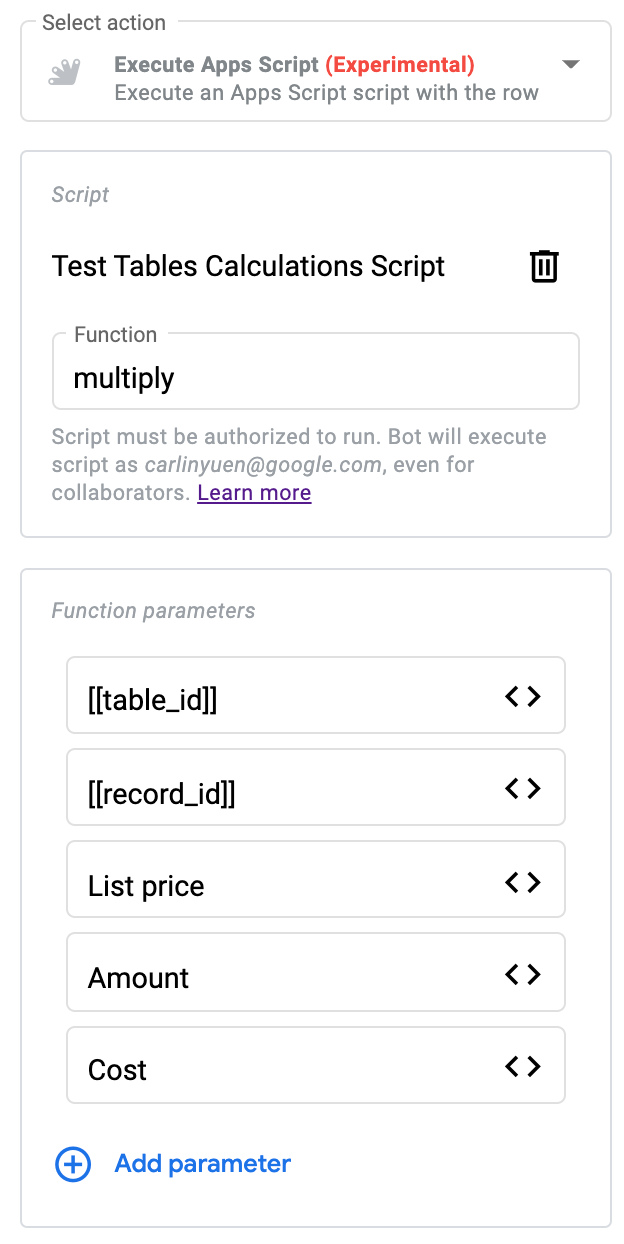NOTE: the order of the function parameters you list in the bot matters, and needs to match up with the function definition in the script.

### Debugging & troubleshooting

If your bot isn't working like you expect, here are some steps to determine what might be going wrong:

## Check that the bot is enabled and set with the correct triggers:

• For time-based: if no bot conditions/filters are set, then the bot may trigger for every row.
• For column-changed: if the trigger column is deleted/changes type, you'll need to reconfigure the trigger.

## If using an "email"-type bot action:

• Make sure recipients are set correctly, they need to be valid email addresses, or Google accounts (via a Person-type column).
• Make sure email subject and body are valid and don't have incorrect use of variables.
• You can test that emails are being sent correctly by manually entering your own email address first.

## If using an "add/update row"-type bot action:

• Make sure any values set are correct for the destination column type.
• Try "clearing" the value and re-setting the values that are not carrying through or may have been using variables from columns that were deleted or had column type changed.

## If using an "execution Apps Script" bot action:

• Make sure the correct script is selected and the correct parameters are passed in the correct order.
• Make sure the bot execution history to see if there were any errors or failure messages: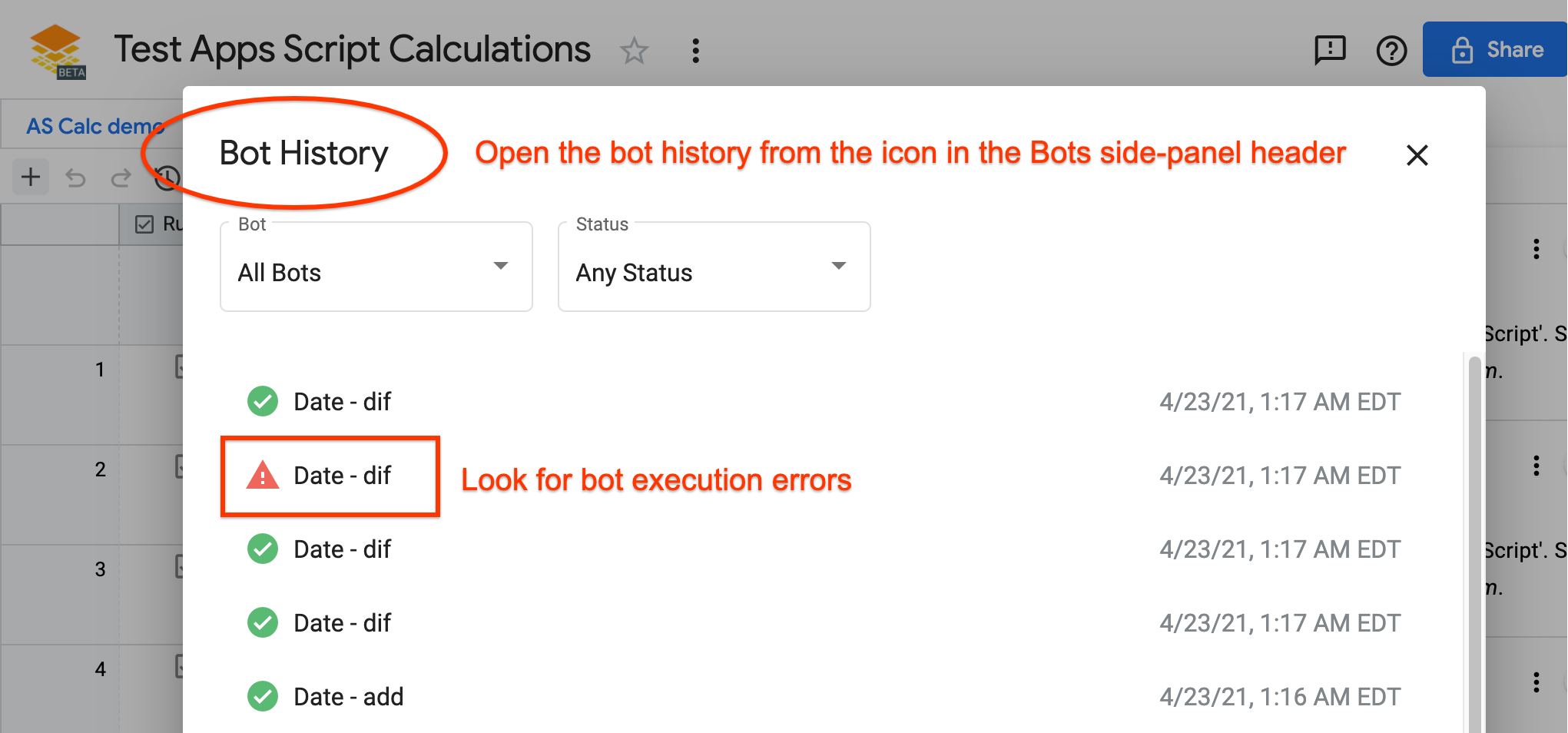• Make sure the Apps Script execution history for errors and debugging messages: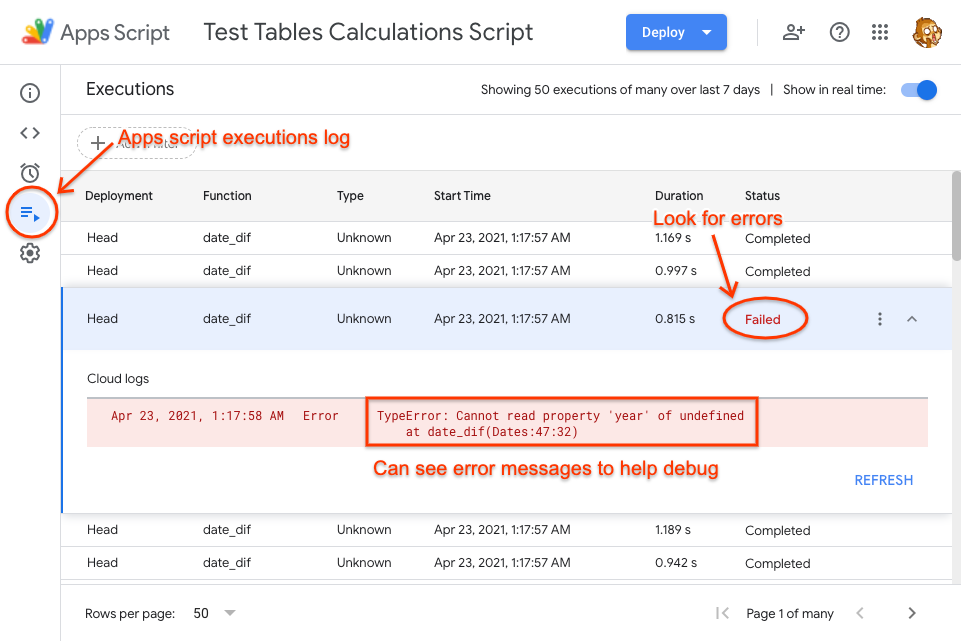• Try other common troubleshooting steps for Apps Script, and reach out to the larger public Apps Script community, which is a great resource for quick questions and answers from other community experts.

Search
Clear search
Close search